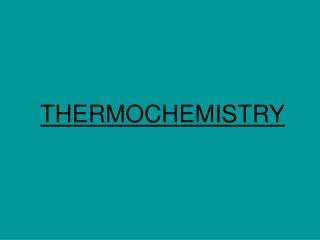DownloadDownload PresentationTHERMOCHEMISTRY

# THERMOCHEMISTRY

Download Presentation## THERMOCHEMISTRY

- - - - - - - - - - - - - - - - - - - - - - - - - - - E N D - - - - - - - - - - - - - - - - - - - - - - - - - - -
##### Presentation Transcript

1. THERMOCHEMISTRY

2. Definitions #1 Energy: The capacity to do work or produce heat Potential Energy: Energy due to position or composition Kinetic Energy: Energy due to the motion of the object

3. Definitions #2 Law of Conservation of Energy: Energy can neither be created nor destroyed, but can be converted between forms The First Law of Thermodynamics: The total energy content of the universe is constant

4. State Functions depend ONLY on the present state of the system ENERGYIS A STATE FUNCTION A person standing at the top of Mt. Everest has the same potential energy whether they got there by hiking up, or by falling down from a plane! WORKIS NOT A STATE FUNCTION WHY NOT???

5. E = q + w E = change in internal energy of a system q = heat flowing into or out of the system -q if energy is leaving to the surroundings +q if energy is entering from the surroundings w = work done by, or on, the system -w if work is done by the system on the surroundings +w if work is done on the system by the surroundings

6. Work, Pressure, and Volume Compression Expansion +V (increase) -V (decrease) -w results +w results Esystemdecreases Esystemincreases Work has been done on the system by the surroundings Work has been done by the system on the surroundings

7. Energy Change in Chemical Processes Endothermic: Reactions in which energy flows into the system as the reaction proceeds. + qsystem - qsurroundings Exothermic: Reactions in which energy flows out of the system as the reaction proceeds. - qsystem + qsurroundings

8. Endothermic Reactions

9. Exothermic Reactions

10. Calorimetry The amount of heat absorbed or released during a physical or chemical change can be measured… …usually by the change in temperature of a known quantity of water 1 calorie is the heat required to raise the temperature of 1 gram of water by 1 C 1 BTU is the heat required to raise the temperature of 1 pound of water by 1 F

11. The Joule The unit of heat used in modern thermochemistry is the Joule 1 joule = 4.184 calories

12. A Bomb Calorimeter

13. A Cheaper Calorimeter

14. Specific Heat The amount of heat required to raise the temperature of one gram of substance by one degree Celsius.

15. Calculations Involving Specific Heat OR s = Specific Heat Capacity q = Heat lost or gained T = Temperature change

16. Hess’s Law “In going from a particular set of reactants to a particular set of products, the change in enthalpy is the same whether the reaction takes place in one step or a series of steps.”

17. Hess’s Law

18. Hess’s Law Example Problem CH4 C + 2H2 +74.80 kJ Step #1: CH4 must appear on the reactant side, so we reverse reaction #1 and change the sign on H.

19. Hess’s Law Example Problem CH4 C + 2H2 +74.80 kJ C + O2 CO2 -393.50 kJ Step #2: Keep reaction #2 unchanged, because CO2 belongs on the product side

20. CH4 C + 2H2 +74.80 kJ C + O2 CO2 -393.50 kJ 2H2 + O2 2 H2O -571.66 kJ Step #3: Multiply reaction #2 by 2

21. Hess’s Law Example Problem CH4 C + 2H2 +74.80 kJ C + O2 CO2 -393.50 kJ 2H2 + O2 2 H2O -571.66 kJ CH4 + 2O2 CO2 + 2H2O -890.36 kJ Step #4: Sum up reaction and H

22. Calculation of Heat of Reaction from heat of formation Hrxn = Hf(products) - Hf(reactants) Hrxn = [-393.50kJ + 2(-285.83kJ)] – [-74.80kJ] Hrxn = -890.36 kJ